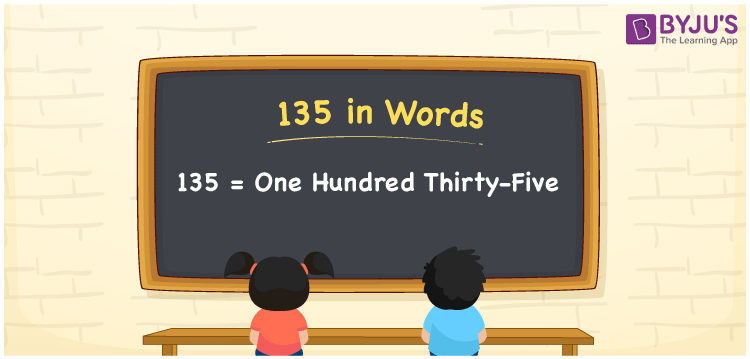# 135 in Words

135 in words is written as One Hundred Thirty-five. The name of number 135 in English is “One Hundred Thirty-five”. The word One Hundred Thirty-five is used in many real-life situations. For example, there are One Hundred Thirty-five students in a playground. Thus, it is a counting number. Also, to represent the currency equal to 135, we can write it in words as Rupees One Hundred Thirty-five or One Hundred Thirty-five rupees. Therefore, it is necessary to learn the numbers in words, for the ease of understanding and expressing them.

 135 in words One Hundred Thirty-five One Hundred Thirty-five in Numerals 135

## 135 in English Words## How to Write 135 in Words?

If we know the place value of digits of 135, then we can easily express it in words. The place value is basically the position of a digit in a number. 135 is a three-digit number, therefore, we can specify the position of each digit of 135 in a place value chart. In the Indian numbering system, the order of place value of digits from right to left is given by:

 Hundreds Tens Ones 1 3 5

We can write it in expanded form as

1 × Hundred + 3 × Ten + 5 × One

= 1 × 100 + 3 × 10 + 5 × 1

= 100 + 30 + 5

= 135

= One Hundred Thirty-five

Therefore, 135 in words is written as One Hundred Thirty-five.

135 is a natural number that precedes 136 and succeeds 134.

135 in words – One Hundred Thirty-five

Is 135 an odd number? – Yes

Is 135 an even number? – No

Is 135 a perfect square number? – No

Is 135 a perfect cube number? – No

Is 135 a prime number? – No

Is 135 a composite number? – Yes

## Frequently Asked Questions on 135 in Words

Q1

### How to write 135 in words?

135 in English is written as “One Hundred Thirty-five”.
Q2

### Is 135 an odd number or even number?

135 is an odd number because it is not wholly divisible by 2.
Q3

### Write One Hundred Thirty-five in numbers.

One Hundred Thirty-five in numbers is 135.
Test your Knowledge on 135 in Words